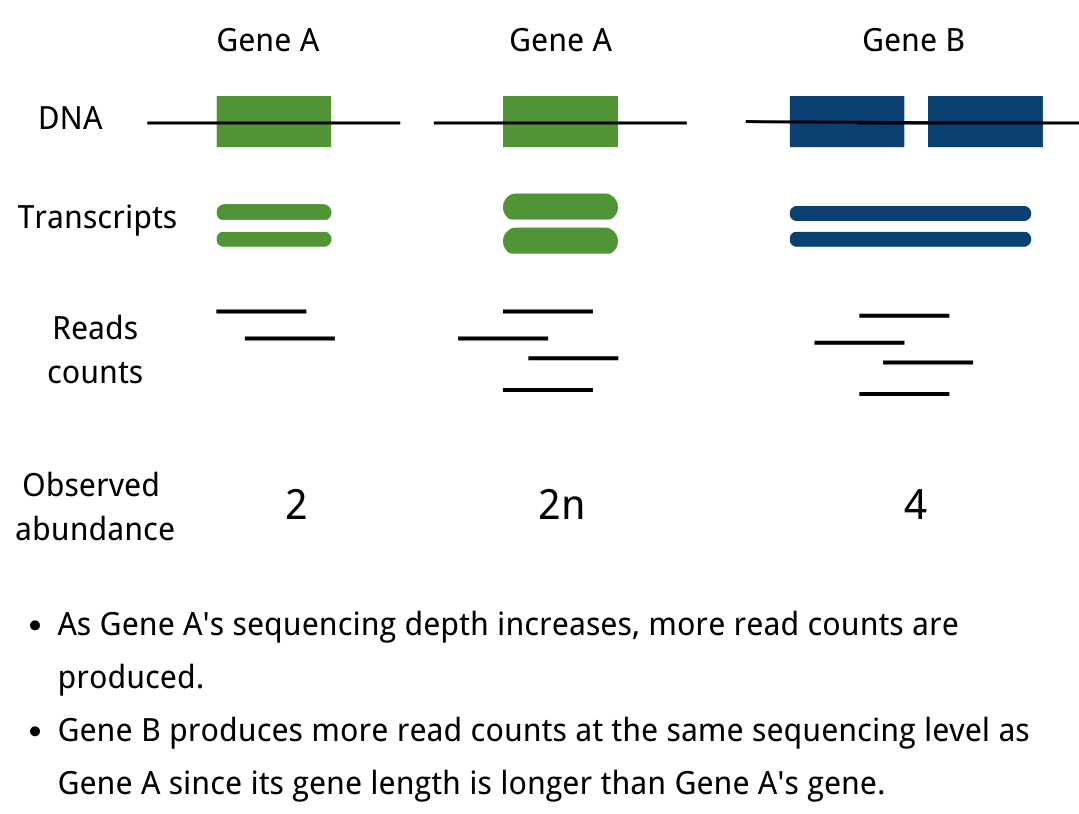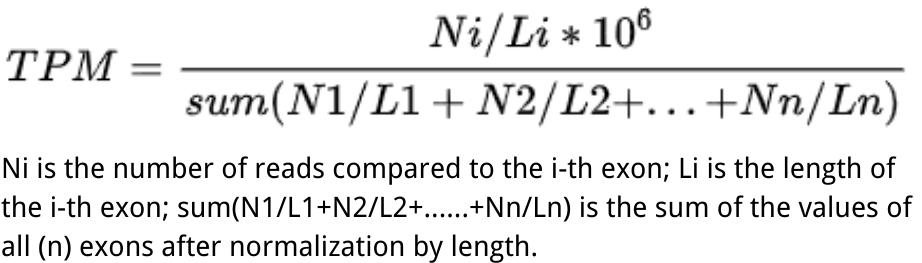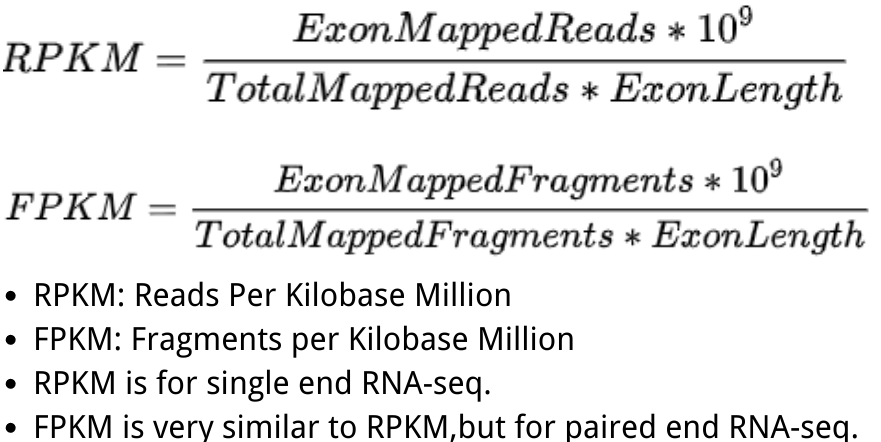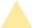• Language
Request A Quote
Featured Blog

## How to choose Normalization methods (TPM/RPKM/FPKM) for mRNA expression

##### Why do mRNA expression values need to be normalized?

The unification of mRNA expression value measurements across studies, or the normalization of mRNA data, is a significant problem in biomedical and life science research. The abundance of transcripts is measured digitally by reading count. To eliminate technical biases in sequenced data, such as sequencing depth(deeper sequencing depth produces more read counts for one gene) and gene length(longer gene length produces more read counts at the same sequencing level), normalization of gene expression measurements is required.Notes:
● READS COUNTS: Obtained from the original sequencing data, the count number is the total number of reads mapped to a certain gene; in the sequencing analysis process, the measured short reads are firstly mapped to the reference genome, and then the software is used to calculate the number of reads mapped to a certain gene, which means that read count is an integer value.

RPKM (Reads Per Kilobase per Million mapped reads）was made for single-end RNA-seq, where every read corresponded to a single fragment that was sequenced. FPKM (Fragments Per Kilobase per Million mapped fragments) is very similar to RPKM. We divide the number of fragments of a gene by the total sequencing depth, and the ratio is divided by the gene length. Note that, strictly speaking, the gene length mentioned above represents the total length of exons from one gene.

The difference between RPKM and FPKM is that F stands for fragments and R stands for reads. In the case of PE (Pair-end) sequencing, each fragment will have two reads, and FPKM only calculates the number of fragments that can be compared to the same transcript for both reads, while RPKM calculates the number of reads that can be compared to the transcript. The FPKM only counts the number of fragments that can be matched to the same transcript. In the case of SE (single-end) sequencing, the results calculated by FPKM and RPKM will be the same.

FPKM and RPKM ultimately normalize the abundance of transcripts from different samples (or the same sample under different conditions) to a standard that allows quantitative comparison by dividing both L (transcript length) and N (total number of Reads (Fragment)).

TPM (transcripts per kilobase million) is very much like FPKM and RPKM, but the only difference is that at first, normalize for gene length, and later normalize for sequencing depth. However, the differencing effect is very profound. Therefore, TPM is a more accurate statistic when calculating gene expression comparisons across samples. While using TPM, the sum of all TPMs are the same in each sample. This makes the comparison of the proportion of reads mapped to a gene in each sample very convenient.##### How to choose the normalization method?

The TPM normalization results are sample independent and the TPMs are guaranteed to be the same across samples; however, the FPKM and TPM are about the same for each gene in each sample, so many people still use FPKM or RPKM to compare expression values of the same gene across samples. As with any high sequencing throughput technology, the analytical method is critical to interpret the data, and the RNA-seq analysis process is always evolving. Therefore, the appropriate method should be selected based on a combination of research directions.

 Normalization method Description Recommendations for use TPM (transcripts per kilobase million) Counts per length of transcript (kb) per million reads mapped when comparing the expression of different genes within a sample. RPKM/FPKM (reads/fragments per kilobase per million reads/fragments mapped) Normalize for gene length at first, and later normalize for sequencing depth when comparing regions within a sample

Reference

1. Dillies, Marie-Agnès, et al. “A comprehensive evaluation of normalization methods for Illumina high-throughput RNA sequencing data analysis.” Briefings in bioinformatics 14.6 (2013): 671-683.
2. 2.Fundel, K., et al. “Normalization strategies for mRNA expression data in cartilage research.” Osteoarthritis and cartilage 16.8 (2008): 947-955.

## More Resources